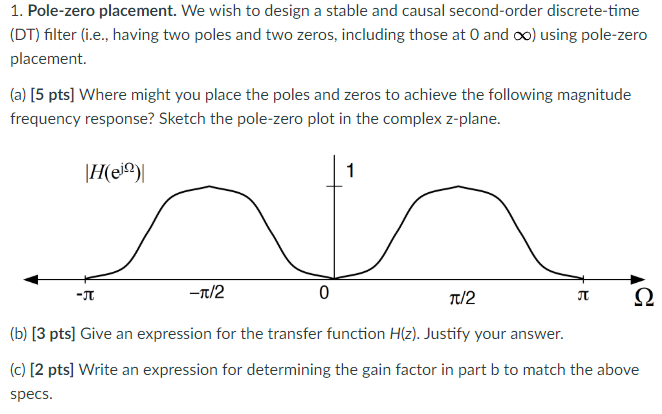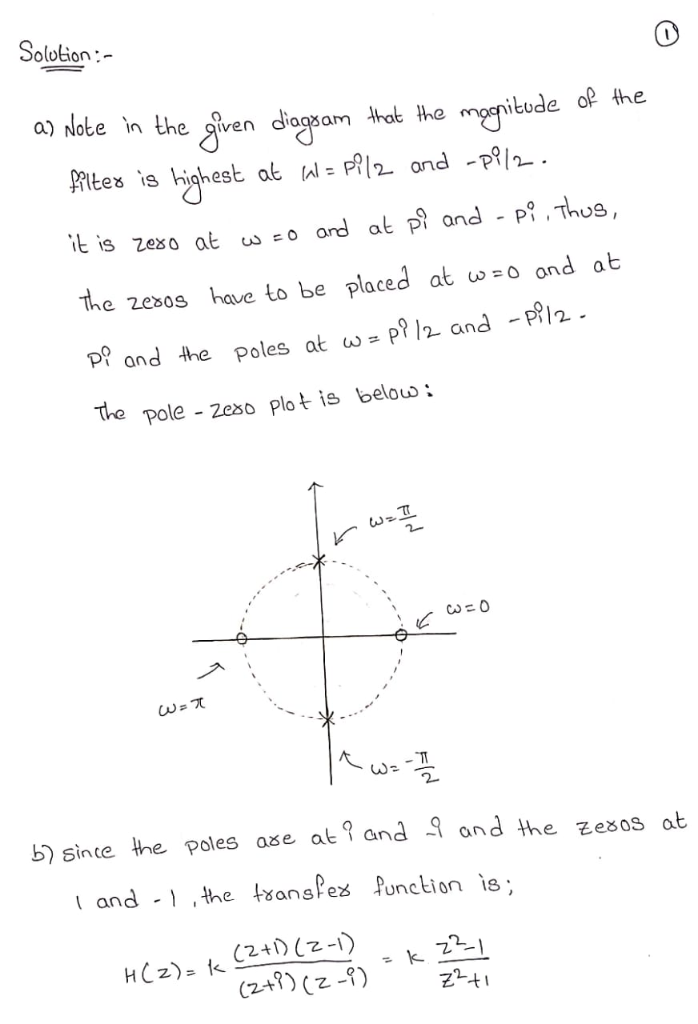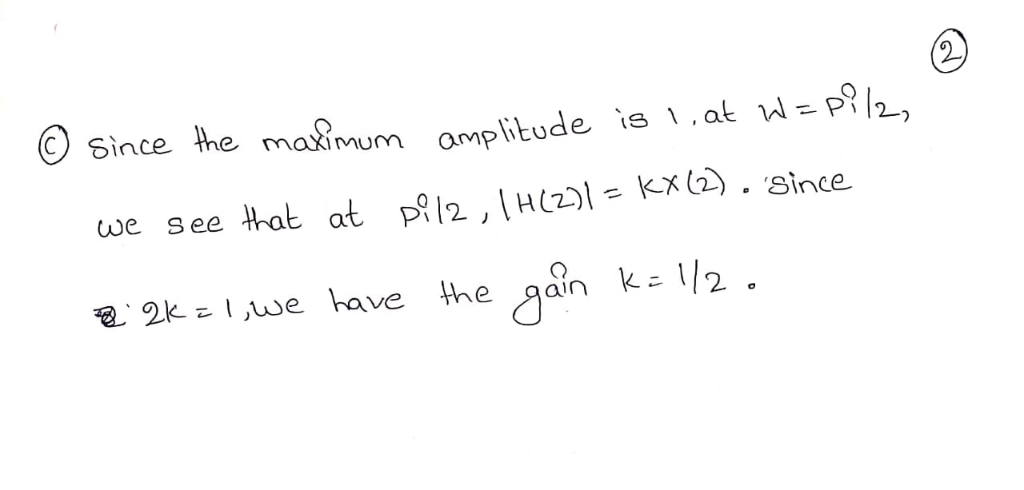Homework Help Question & Answers

# 1. Pole-zero placement. We wish to design a stable and causal second-order discrete-time (DT) fil...1. Pole-zero placement. We wish to design a stable and causal second-order discrete-time (DT) filter (i.e., having two poles and two zeros, including those at 0 and oo) using pole-zero placement. (a) [5 pts] Where might you place the poles and zeros to achieve the following magnitude frequency response? Sketch the pole-zero plot in the complex z-plane. -Π -Tt/2 0 (b) [3 pts] Give an expression for the transfer function H(z). Justify your answer. (c) [2 pts] Write an expression for determining the gain factor in part b to match the above specs

#### Homework Answers

ReportAnswer #1Add Homework Help Answer
##### Add Answer of: 1. Pole-zero placement. We wish to design a stable and causal second-order discrete-time (DT) fil...
Your Answer: Your Name: What's your source?
Not the answer you're looking for? Ask your own homework help question. Our experts will answer your question WITHIN MINUTES for Free.
More Homework Help Questions Additional questions in this topic.

• #### A discrete-time LTI system has the system function H(z) given below: 2 H(z (a) Sketch the pole-zero plot for this system. How many possible (ROCs) are there for H(z). List the possible ROCs and indic...

Need Online Homework Help?

Get FREE EXPERT Answers
WITHIN MINUTES
Related Questions